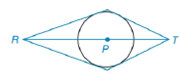Chapter 6.4, Problem 16EElementary Geometry For College St...

7th Edition
Alexander + 2 others
ISBN: 9781337614085

Solutions

Chapter
SectionElementary Geometry For College St...

7th Edition
Alexander + 2 others
ISBN: 9781337614085
Textbook Problem

Given circle P and points R − P − T such that R and T are in the exterior of circle P , suppose that tangents are constructed from R and T to form a quadrilateral (as shown). Identify the type of quadrilateral formeda) when R P > P T .b) when R P = P T .To determine

(a)

To identify:

The type of quadrilateral formed based on the condition when RP>PT.

Explanation

A quadrilateral in which two pairs of adjacent sides are equal and diagonals intersect at 90° and only one diagonal is bisected by the other is called a kite. Thus, in a kite AB=AD and BC=CD. Also, AEB=AED=BEC=DEC=90°.

The line that is perpendicular to the radius of a circle at its endpoint on the circle is a tangent to the circle. The tangents from an exterior point to the circle are two in numbers and the length of both is same at the point of intersection of the circle. In the figure below from point S two tangents are drawn touching the circle at R and T,

So, SR=ST

To determine

(b)

To identify:

The type of quadrilateral formed based on the condition when, RP=PT.

Still sussing out bartleby?

Check out a sample textbook solution.

See a sample solution

The Solution to Your Study Problems

Bartleby provides explanations to thousands of textbook problems written by our experts, many with advanced degrees!

Get Started

Solve the equations in Exercises 126. 10x(x2+1)4(x3+1)510x2(x2+1)5(x3+1)4=0

Finite Mathematics and Applied Calculus (MindTap Course List)

Finding Derivatives In Exercises 51-62, find f(x). f(x)=x4/5+x

Calculus: An Applied Approach (MindTap Course List)

What is the lowest score in the following distribution?

Essentials of Statistics for The Behavioral Sciences (MindTap Course List)

In Exercises 19-24, find the functions f + g, f g, fg, and fg. 24. f(x) = x2 + 1; g(x) = x+1

Applied Calculus for the Managerial, Life, and Social Sciences: A Brief Approach

The point P graphed at the right has spherical coordinates:

Study Guide for Stewart's Multivariable Calculus, 8th